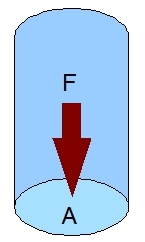# Pressure

p = F / A = dF / dA

where:
p : Pressure (Pascal or N/m2)
F : Normal force (N)
A : Area (m2)## Explanation

Pressure is a scalar quantity. Pressure is an effect which occurs when a force is applied on a surface. Pressure is transmitted to solid boundaries or across arbitrary sections of fluid normal to these boundaries or sections at every point.

## Example

The pressure of air at the surface of the earth is caused by the weight of the air column. A column of air with a cross section of 1 m2 has an approximate mass of 10200 kg.

P = F / A = mg / A = 10200 . 9.8 / 1 = 99960 N/m2 (or 99.96 kP)

# Do you want to comment this topic?

1: (Book) Cyclic and Collective2: (Book) Principles of Helicopter Flight4: Logitech Extreme 3D Pro Joystick5: Saitek Pro Flight Rudder Pedals